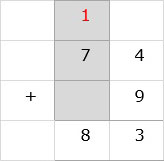# Adding 2-digit Numbers and Single Digit Numbers

#### Excel add-in with c# VSTO and web

7 Lectures 1.5 hours

#### Word add-in using c# vsto and web types

8 Lectures 1 hours

#### The Ultimate Guide To Add Social Media Login to PHP Web Apps

17 Lectures 2 hours

Add the numbers 57 + 8

### Solution

Step 1:

We line up the numbers vertically.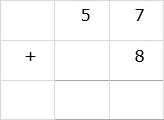Step 2:

We add the digits in ones column. 7 + 8 = 15. 5 is written below ones column and 1 is carried over to tens column.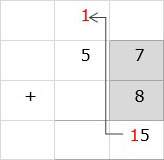Step 3:

In tens column, 5 + 1 = 6. We write 6 below tens column. So the final sum is 65.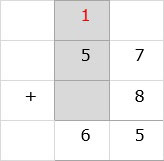Add the numbers 74 + 9

### Solution

Step 1:

We line up the numbers vertically.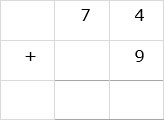Step 2:

We add the digits in ones column. 4 + 9 = 13. 3 is written below ones column and 1 is carried over to tens column.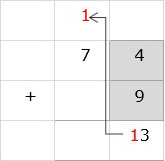Step 3:

In tens column, 7 + 1 = 8. We write 8 below tens column. So the final sum is 83.